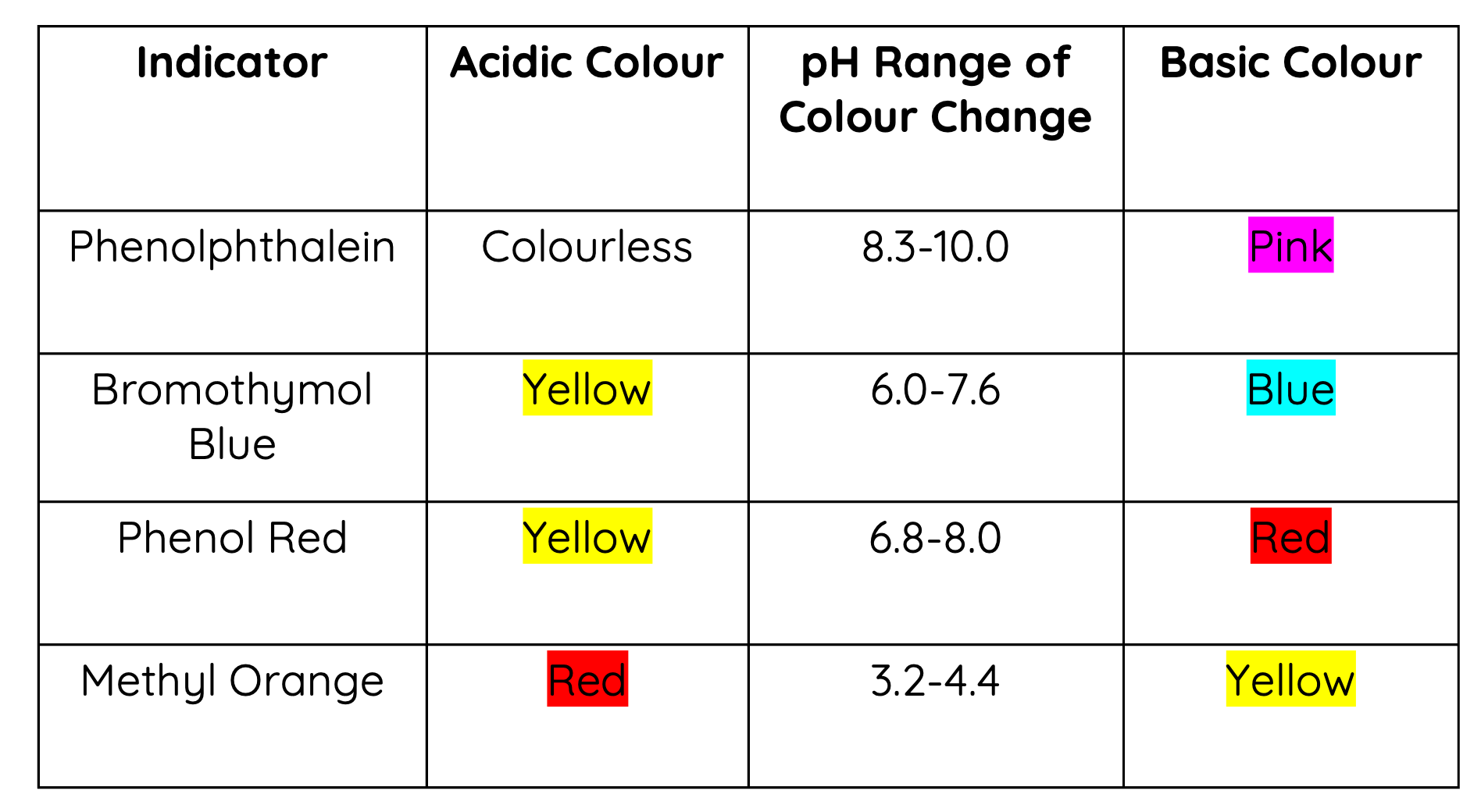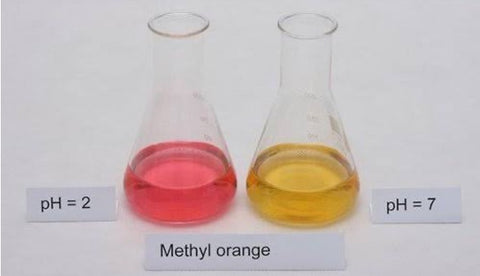# pH Indicators

This is part of the HSC Chemistry course under the topic Properties of Acids and Bases.

### HSC Chemistry Syllabus

• Conduct an investigation to demonstrate the preparation and use of indicators as illustrators of the characteristics and properties of acids and bases and their reversible reactions (ACSCH101)

### pH Indicators

Indicators are chemicals that can be added to a solution to determine its acidity or basicity.

This video discusses some common examples of pH indicators including

• Methyl orange
• Bromothymol blue
• Phenolphthalein

### What are pH Indicators?

pH indicators are chemicals that provide qualitative information on a solution's pH. They change colour as the pH of a solution changes.

pH indicators are used in acid/base titration to determine the concentration of a solution of acid or base.

### Common pH Indicators### How to Use Indicators

Multiple pH indicators should be used to estimate the pH of a solution. Using a single indicator will only provide a range for pH.

For example, a solution is tested with four indicators separately. The observation is shown in the table.The solution appears green when tested with bromothymol blue. Since green is the transition colour between yellow and blue, this implies the solution's pH lies between 6.0 and 7.6.

The solution appears orange when tested with phenol red. Since orange is the transition colour between red and yellow, this implies the solution's pH lies between 6.8 and 8.0.

Combining the results above, the solution's pH lies between 6.8 and 7.6.

The observation made from phenolphthalein and methyl orange are not helpful as it does not further narrow down the pH range of estimation.

### How do Indicators Work?

pH indicators are weak acids. When an indicator is added to a solution, it reaches an equilibrium with its conjugate base. HIn represents the protonated form of the indicator and In^- represents the deprotonated form.

$$HIn(aq) + H_2O(l) \rightleftharpoons In^-(aq) + H_3O^+(aq)$$

A particular pH indicator will appear one colour when protonated and change colour when it is deprotonated. The relative concentrations of HIn and In^- determines the colour of the solution.

As the ph a solution changes, the concentration of hydrogen (hydronium) ions changes. According to Le Chatelier's principle, this change will cause the equilibrium position to shift in order to counteract the change.

When [H_3O^+] increases (lower pH), the equilibrium will shift to the left. As a result, the concentration of the protonated form of the indicator, [HIn] will increase. The colour of the solution will change such that it will appear more like the colour of HIn.

When [H_3O^+] decreases (higher pH), the equilibrium will shift to the right. As a result, the concentration of the deprotonated form of the indicator, [In^-] will increase. The colour of the solution will change such that it will appear more like the colour of In^-.

### Strength of Indicators

pH indicators change colour at different pH values because they have varying degree of ionisation (strength). The pKa of an indicator is the pH at which it becomes 50% ionised (deprotonated). When an indicator is 50% ionised, [HIn] and [In^-] are equal, and therefore the colour of the solution will be an even mix of the colours of HIn and In^-.For example, the pKof phenolphthalein is 9.4. This means at pH = 9.4, a solution with phenolphthalein will change colour between pink and colourless. This is consistent with the pH range of colour change for phenolphthalein (8.3-10.0).The pKof methyl orange is 3.4. This means at pH = 3.4, a solution with methyl orange will appear orange (transition between yellow and red). This is consistent with the pH range of colour change for methyl orange (3.1-4.4).